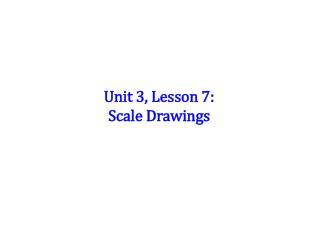DownloadDownload PresentationUnit 3, Lesson 7: Scale Drawings

# Unit 3, Lesson 7: Scale Drawings

Télécharger la présentation## Unit 3, Lesson 7: Scale Drawings

- - - - - - - - - - - - - - - - - - - - - - - - - - - E N D - - - - - - - - - - - - - - - - - - - - - - - - - - -
##### Presentation Transcript

1. Unit 3, Lesson 7: Scale Drawings

2. Scale drawings are used to represent objects that are either too large or too small for a life size drawing to be useful. Examples: A life size drawing of an ant or an atom would be too small to be useful. A life size drawing of the state of Michigan or the Solar System would be too large to be useful.

3. A scale is always provided with a scale drawing. The scaleis the ratio: drawing real life (actual) When solving a problem involving scale drawings you should: Write the scale as a ratio. Write the second ratio by putting the provided information in the correct location (drawing in numerator & real life in denominator). Solve the proportion.

4. Example: This drawing has a scale of "1:10", so anything drawn with the size of "1" would have a size of "10" in the real world, so a measurement of 150mm on the drawing would be 1500mm on the real horse.

5. Example: The distance between Philadelphia and San Francisco is 2,950 miles. You look on a map and see the scale is 1 inch : 100 miles. What is the distance between the two cities on the map?

6. Example: On a map, the distance between your town and Washington DC is 3.6 inches. The scale is 1 inch : 55 miles. What is the distance between the two cities?

7. On a map, the scale is 1/2 inch= 300 miles. Find the actual distance between two stores that are 5 1/2 inches apart on the map. 3000 miles A B 2,727 miles 3,300 miles C 1,650 miles D

8. The figure is a scale of the east side of a house. In the drawing, the side of each square represents 4 feet. Find the width and height of the door. 4 ft by 9 ft A B 4 ft by 12 ft 4 ft by 8 ft C D 4 ft by 10 ft

9. On a map with a scale of 1 inch =100 miles, the distance between two cities is 7.55 inches. If a car travels 55 miles per hour, about how long will it take to get from one city to the other. 13 hrs 45 min. A B 14 hrs 30 min. 12 hrs C D 12 hrs 45 min.